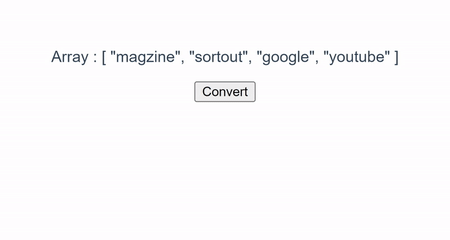# How to convert array to string in VueJS?

Hi Friends 👋,

Welcome To SortoutCode! ❤️

To convert array to string in VueJS. There are different ways to convert the array to a string. In this, We are going to use the `toString()` and `join()` methods to convert the array data into a string. We are also going to use the `for loop` method to array data to a string.

## Short solution

`parentComp.vue`

``````var Arry = ['Monday',' Tuesday','Sunday']; //Array

// using the toString() method
arrA = Arry.toString();

console.log(arrA); // 'Monday, Tuesday,Sunday'

// using the join() method
arrB = Arry.join(',');

console.log(arr); // 'Monday, Tuesday,Sunday'

let arrC = '';
for (let i = 0; i < Arry.length; i++) {
arrC += Arry[i];
if (i !== Arry.length - 1) {
arrC += ',';
}
}
return str;

console.log(arrC);  // 'Monday,Tuesday,Sunday'``````

Today, I am going to show you How you convert an array to string in VueJS?

Table of Content

• Setup the Vue (Optional)
• Create FirstComponent.vue and import it into App.js
• Convert array to string using toString() method
• Convert array to string using join() method
• Convert array to string using for loop

This article will guide you to How do I convert array to string in vueJS

## Setup the Vue.js

First, we have to install the Vue project, I had installed the vueJS in my system. If you haven’t installed or have any problem with installation you can follow this article, it will show you step by step process of installation.

How to Install the VueJS project?

## Create FirstComponent.vue and import it into App.js

Create the new component in the `src/components/` components folder. The component name is `FirstComponent.vue` and imported into the `App.js` file:

`App.js`

``````<template>
<FirstComp />
</template>

<script>
import FirstComp from "./components/FirstComp.vue";
export default {
name: "App",
components: {
FirstComp: FirstComp,
},
};
</script>``````

## Convert array to string using toString() method

To convert the array to string in vuejs. We are going to define the array `ary` in the data property. And we are going to convert this array into a string using the `toString()` method. This method will convert the array to a string with a comma-separated string.

Let’s take a short example to better understand.

`FirstComp.vue`

``````<template>
<div class="hello">
<p>Array : {{ ary }}</p>
<p v-if="output">string : {{ output }}</p>
<p v-if="output">DataType is : {{ typeof output }}</p>
<button @click="convertData">Convert</button>
</div>
</template>

<script>
export default {
name: "FirstComp",
data() {
return {
output: "",
};
},
methods: {
convertData() {
this.output = this.ary.toString();
},
},
};
</script>``````

For now, let’s check the output.

## Output## Convert array to string using join() method

To convert the array to string in vuejs. We are going to define the array `ary` in the data property. And we are going to convert this array into the string using the `join()` method. This method will convert the array to a string with a comma-separated string.

Let’s take a short example to better understand.

`FirstComp.vue`

``````<template>
<div class="hello">
<p>Array : {{ ary }}</p>
<p v-if="output">string : {{ output }}</p>
<p v-if="output">DataType is : {{ typeof output }}</p>
<button @click="convertData">Convert</button>
</div>
</template>

<script>
export default {
name: "FirstComp",
data() {
return {
output: "",
};
},
methods: {
convertData() {
this.output = this.ary.join(',');
},
},
};
</script>``````

For now, let’s check the output.

## Output## Convert array to string using for loop

To convert the array to string in vuejs. We are going to define the array `ary` in the data property. And we are going to convert this array into the string using the `for loop`. This method will convert the array to a string with a comma-separated string.

Let’s take a short example to better understand.

`FirstComp.vue`

``````<template>
<div class="hello">
<p>Array : {{ ary }}</p>
<p v-if="output">string : {{ output }}</p>
<p v-if="output">DataType is : {{ typeof output }}</p>
<button @click="convertData">Convert</button>
</div>
</template>

<script>
export default {
name: "FirstComp",
data() {
return {
output: "",
};
},
methods: {
convertData() {
for (let i = 0; i < this.ary.length; i++) {
this.output += this.ary[i];
if (i !== this.ary.length - 1) {
this.output += ",";
}
}
},
},
};
</script>``````

For now, let’s check the output.

## OutputHere, is the above program code sandbox link of how to convert an array to a string using the `toString()`, `join()`, and `for loop` of these three methods in vue js. Then you can use it whenever you went and do the changes as per your requirements.